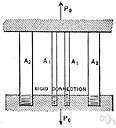# modulus of elasticity

(redirected from Chord modulus)
Also found in: Thesaurus, Medical, Encyclopedia, Wikipedia.

## modulus of elasticity

n
(General Physics) the ratio of the stress applied to a body or substance to the resulting strain within the elastic limit. Also called: elastic modulus See also Young's modulus, bulk modulus, modulus of rigidity
Collins English Dictionary – Complete and Unabridged, 12th Edition 2014 © HarperCollins Publishers 1991, 1994, 1998, 2000, 2003, 2006, 2007, 2009, 2011, 2014
ThesaurusAntonymsRelated WordsSynonymsLegend:
 Noun 1modulus of elasticity - (physics) the ratio of the applied stress to the change in shape of an elastic bodynatural philosophy, physics - the science of matter and energy and their interactions; "his favorite subject was physics"modulus - (physics) a coefficient that expresses how much of a specified property is possessed by a specified substancebulk modulus - the ratio of the change in pressure acting on a volume to the fractional change in volumemodulus of rigidity - the coefficient of elasticity for a shearing forceYoung's modulus - a coefficient of elasticity applicable to the stretching of a wire
Based on WordNet 3.0, Farlex clipart collection. © 2003-2012 Princeton University, Farlex Inc.
Translations
modulo di elasticità
References in periodicals archive ?
There are three ways to calculate the elastic modulus: (1) the tangent modulus, (2) the secant modulus, and (3) the chord modulus. The static compressive elastic modulus studied in this experiment is actually the concrete's chord modulus.
The strain results are used for chord modulus and Poisson's ratio  calculations necessary to develop, integrate and implement predictive computational models for CFRPs composites linking the materials design, molding process and final performance requirements for optimal design and manufacturing of CFRP automotive systems.
From tensile tests, ultimate tensile strength, strain at maximum load (as defined in ISO 527-1 Standard) and tensile chord modulus of elasticity (Young's modulus) were calculated; a strain range of 0.003 to 0.005 mm/mm was used for chord modulus calculation.

Site: Follow: Share:
Open / Close